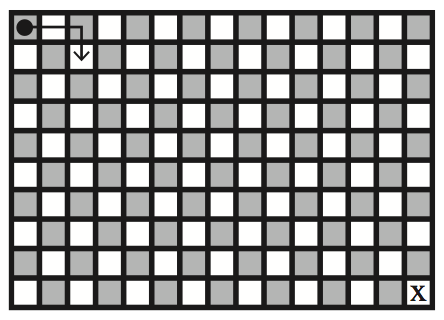#### Programming

###### back to index

Initially, a chip is placed in the upper-left corner square of a $n\times m$ grid of squares as shown. The chip can move in an $L$-shaped pattern, moving two squares in one direction (up, right, down or left) and then moving one square in a corresponding perpendicular direction. What is the minimum number of $L$-shaped moves needed to move the chip from its initial location to the square marked “$X$”?Find the smallest square which can cover $n$ congruent equilateral triangles so that these triangles do not overlap.

Determine if one binary tree is a sub-tree of a larger one.

Generate all balanced bracket combinations. For example, when $N=2$, the output should be $(())$, $()()$.